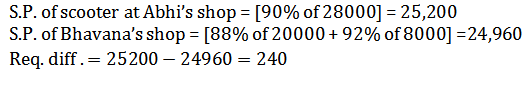## Important Quantitative Aptitude Questions For SSC CGL 2018

Quantitative Aptitude Questions For SSC CGL 2018. Welcome to the www.letsstudytogether.co online SSC CGL 2018 online preparation section. If you are preparing SSC CGL, CPO Exams 2018, you will come across a section on “Quantitative Aptitude “. Here we are providing you with “Important Quantitative Aptitude Questions For SSC CGL Prelims 2018”   based on the latest pattern of your daily practice.

This “Quantitative Aptitude Questions For SSC CGL 2018“ is also important for other SSC & Railway exams such as CPO, CHSL, RRB Group D, ALP & Technicians Exam 2018.

## Important Quantitative Aptitude Questions For SSC CGL | Set -1

1.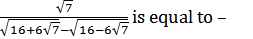A. 1/2

B. 1/3

C. 1/4

D. 1/5

A. 1/2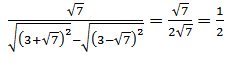2. When a certain positive integer P is divided by another positive integer, the remainder is r1, when a second positive integer Q is divided by the same integer, the remainder is r2, and when (P+Q) is divided by the same divisor, the remainders is r3 Then the divisor may be

A. (r1+r2+r3)

B. (r1– r2+r3)

C. (r1+r2-r3)

D. (r1r2r3)

C. (r1+r2-r3)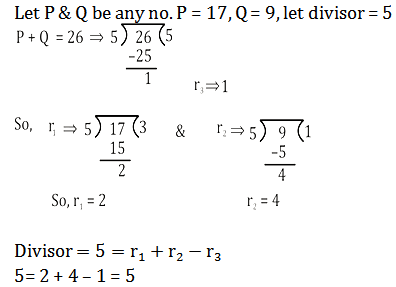3.In a set of 5 numbers the sum of two of these numbers is 6 more than the sum of the remaining three numbers, whereas the sum of these three numbers is two times one of those two numbers. What definitely is one of those two numbers?

A. 18

B. 12

C. 16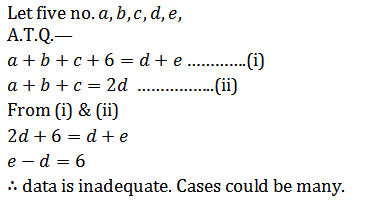4. In Krishna engineering college the average salary of all engineering graduates from mechanical trade is Rs. 2.45 lakhs per annum and that of the engineering graduates from electronic trade is Rs. 3.56 lakhs per annum. The average salary of all mechanical and electronics graduates is Rs. 3.12 lakhs per annum. Find the least number of electronic graduates passing out from this institute.

A. 55

B. 67

C. None of these

D. Cannot be determined

B. 67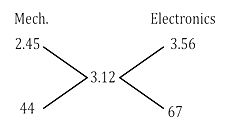Atleast 67 electronic graduates should be there to fulfill the conditions.

5.A solution of Corex cough syrup has 15% sugar. Another solution has 5% sugar. How many liters of the second solution must be added to 20 litres of the first solution to make a solution of 10% sugar?

A. 5 litres

B. 20 litres

C. 16 litres

D. 12 litres

B. 20 litres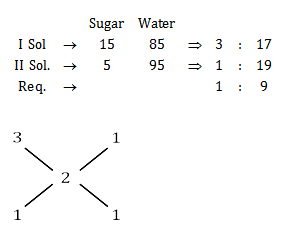So, 20 Ltr. Of 1st solution must be mixed with equal quantity of 2nd solution to make sugar 10% in total mix.

6.1/3 and ¼ parts of two cans of equal volume are filled with milk. The cans are then filled to their capacity with water and the mixture of the two cans are poured in a big pot. The ratio of milk and the water in the pot is –

A. 7 : 17

B. 12 : 13

C. 8 : 11

D. 5 : 13

A. 7 : 17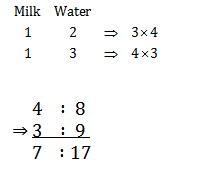7.Seelu started a business investing Rs. 45,000 Navya joined her after six months with Rs. 80,000 and Ramesh joined them with Rs. 1,20,000 after one year. Profit earned at the end of two years after Seelu started the business should be distributed among Seelu, Navya and Ramesh respectively in what ratio?

A. 3 : 4 : 4

B. 4 : 7 : 5

C. 4 : 3 : 3

D. 5 : 7 : 4

A. 3 : 4 : 4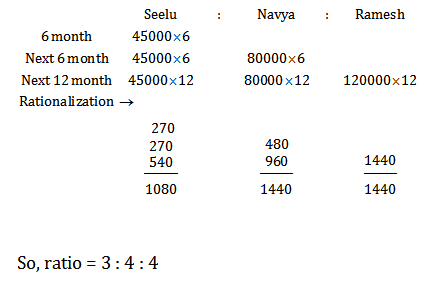8.A person lent out a certain sum on S.I. and the same sum on C.I. at a certain rate of interest p.a. He noticed that the ratio between the difference of C.I. and S.I. in 3 yrs. and 2 yrs. is respectively 25 : 8. The rate of interest is –

A. 25%

B. 12.5%

C. 16.6%

D. 20%

B. 12.5%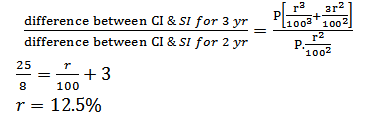9. A 110 meters long train is running with a speed of 60 kmph. In what time will it pass a man who is running at 6 kmph in the direction opposite to that in which the train is going?

A. 6 seconds

B. 10 seconds

C. 7 seconds

D. 9 seconds

A. 6 seconds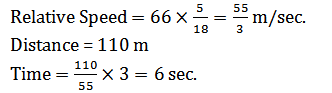10. Abhi and Bhavana both are dealers of LML Scooter. The price of a LML Scooter is Rs. 28,000. Abhishek gives a discount of 10% on whole, while Bhavana gives a discount of 12% on the first Rs. 20,000 and 8% on the rest Rs. 8,000. What is the different between their selling price?

A. Rs. 360

B. Rs. 240

C. Rs. 520

D. Rs. 720

B. Rs. 240